﻿ 高超声速飞行器底层性能评价指标的可行性分析Download PDF文章快速检索 高级检索

1. 南京航空航天大学 自动化学院, 南京 211106;
2. 南京航空航天大学 航天学院, 南京 210016;
3. 北京航空航天大学 虚拟现实技术与系统国家重点实验室, 北京 100083

Feasibility analysis for underlying indictors in control performance evaluation of hypersonic vehicles
ZHU Hongxu1, LIU Yanbin2,3, CAO Rui1, LU Yuping1, TANG Jiajun1, YI Chunlun2
1. College of Automation Engineering, Nanjing University of Aeronautics and Astronautics, Nanjing 211106, China;
2. College of Astronautics, Nanjing University of Aeronautics and Astronautics, Nanjing 210016, China;
3. State Key Laboratory of Virtual Reality Technology and Systems, Beihang University, Beijing 100083, China
Abstract: In order to judge whether the control performance evaluation strategy can be applied to hypersonic vehicles, a feasibility analysis of the bottom parameters is addressed. First, the modeling characteristics of hypersonic vehicles are analyzed, proposing two requirements to make sure that the underlying indictors can be applied to control the performance evaluation of hypersonic vehicles. According to the two requirements, the feasibility analysis is converted to the investigation of the map from mission variables to underlying indictors, and a method based on statistical experiments is proposed to analyze the map. Combined with the characteristics of the hypersonic vehicle model, the flow of the feasibility analysis using the underlying indicators for hypersonic flight mission evaluation is further designed. Then, following the analytic flow, hypersonic flight examples of static mission and command tracking are analyzed, proposing frequency domain indicators and time domain indicators. The simulation is conducted for the first example. Static margin and the restricted elevator deflection are set as mission requirements, while damping and frequency characteristics are set as evaluation parameters. Numerical results indicate that the conclusion of analysis is consistent with the view of military standards, and the analysis flow is relatively easy to implement.
Keywords: hypersonic vehicle    control performance evaluation    feasibility analysis    underlying indictor    statistical analysis

1 高超声速飞行器模型特性分析

1.1 纵向模态特性

1.2 飞行约束

1.3 不确定性

1.4 底层指标可行性的表述

1) 完全覆盖：底层指标应完全覆盖高超声速飞行器包括飞行约束在内的各项控制性能。

2) 抗不确定性扰动：所选择的底层指标受不确定性的干扰应尽可能小。

2 底层指标的可行性分析

2.1 双映射检验方法

 $\frac{{V\left( {{\mathit{\Sigma }_{\rm{a}}} \cap {\mathit{\Sigma }_{\rm{b}}}} \right)}}{{V\left( \mathit{\Sigma } \right)}} = 0$ （1）

f:σΣ是双映射。这里V(*)表示高维空间中封闭区域的体积。

1) 因为Σ是值域，所以f:xy, xσ是满映射。

2) 令ΣaΣb=Σxη=V(Σx)/V(Σ)。对于任意x1, x2σx1x2，存在σa，使得x1σa, x2σb。因为η≡0且η=V(Σx)/V(Σb)，所以Σx=Ø，即f(x2)$\notin$Σa。而f(x1)∈Σa，所以f(x1)≠f(x2)。这样f:xy, xσ是单映射。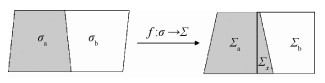图 1 二维线性空间情形下的定理示意图 Fig. 1 Application of theorem in two-dimensional linear space
 $\eta = \frac{{{n_x}}}{{{n_{\rm{t}}}}}$ （2）

2.2 可行性分析流程设计

 $\left\{ \begin{array}{l} \dot v = \frac{{T\cos \alpha - D}}{m} - g\sin \gamma \\ \dot \gamma = \frac{{L + T\sin \alpha }}{{mv}} - \frac{{g\cos \gamma }}{v}\\ \dot h = v\sin \gamma \\ \dot \alpha = q - \dot \gamma \\ \dot q = \frac{M}{{{I_{yy}}}} \end{array} \right.$ （3）

 $\left\{ {\begin{array}{*{20}{l}} {\mathit{\boldsymbol{\dot x}} = \mathit{\boldsymbol{Ax}} + \Delta \mathit{\boldsymbol{u}}}\\ {\Delta \mathit{\boldsymbol{u}} = - \mathit{\boldsymbol{kx}}} \end{array}} \right.$ （4）

 $\left\{ {\begin{array}{*{20}{l}} {\mathit{\boldsymbol{\dot x}} = \left( {\mathit{\boldsymbol{A}} + \Delta \mathit{\boldsymbol{A}}} \right)\mathit{\boldsymbol{x}} + \left( {\mathit{\boldsymbol{B}} + \Delta \mathit{\boldsymbol{B}}} \right)\Delta \mathit{\boldsymbol{u}}}\\ {\Delta \mathit{\boldsymbol{u}} = {\mathit{\boldsymbol{u}}^ * } - \mathit{\boldsymbol{kx}}} \end{array}} \right.$ （5）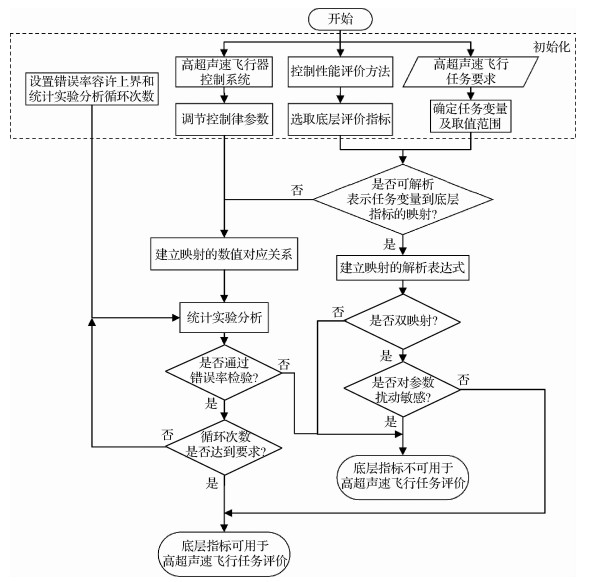图 2 分析流程设计 Fig. 2 Flowchart of analysis

2.3 传统评价指标的可行性分析

 ${\rm{d}}{\delta _{\rm{e}}} = - {K_q}{\rm{d}}q - {K_\alpha }{\rm{d}}\alpha$ （6）

 ${\left( {\partial M/\partial \alpha } \right)_{\rm{b}}} = QS\bar c\left( {{C_{{m_\alpha }}} + {k_T}{C_{{T_\alpha }}}} \right)$ （7）

 ${\left( {\partial M/\partial \alpha } \right)_{{\rm{aug}}}} = QS\bar c\left( {{C_{{m_\alpha }}} - {K_\alpha }{C_{{m_\delta }}} + {k_T}{C_{{T_\alpha }}}} \right)$ （8）

 ${S_{\rm{M}}} = - \frac{{{C_{{m_\alpha }}} - {K_\alpha }{C_{{m_\delta }}} + {k_T}{C_{{T_\alpha }}}}}{{{C_{{L_\alpha }}}}}$ （9）

 ${\delta _{\rm{e}}} = {\delta _{{\rm{e}},{\rm{trim}}}} - {K_q}{\rm{d}}q - {K_\alpha }{\rm{d}}\alpha$ （10）

 $\begin{array}{l} \left[ {\begin{array}{*{20}{c}} {{S_{\rm{M}}}}\\ {{\delta _{\rm{e}}}} \end{array}} \right] = {{f'}^{ - 1}}\left[ {\begin{array}{*{20}{c}} {{K_\alpha }}\\ {{K_q}} \end{array}} \right] = \mathit{\boldsymbol{A'}}\left[ {\begin{array}{*{20}{c}} {{K_\alpha }}\\ {{K_q}} \end{array}} \right] + \mathit{\boldsymbol{B'}} = \\ \;\;\;\;\;\;\;\left[ {\begin{array}{*{20}{c}} {\frac{{{C_{{m_\alpha }}}}}{{{C_{{L_\alpha }}}}}}&0\\ { - \Delta \alpha }&{ - \Delta q} \end{array}} \right]\left[ {\begin{array}{*{20}{c}} {{K_\alpha }}\\ {{K_q}} \end{array}} \right] + \left[ {\begin{array}{*{20}{c}} { - \frac{{{C_{{m_\alpha }}} + {k_T}{C_{{T_\alpha }}}}}{{{C_{{L_\alpha }}}}}}\\ {{\delta _{{\rm{e,trim}}}}} \end{array}} \right] \end{array}$ （11）

 $\kappa \left( {{\mathit{\boldsymbol{A}}^\prime }} \right) = \left\| {{\mathit{\boldsymbol{A}}^\prime }} \right\|\left\| {{\mathit{\boldsymbol{A}}^{\prime - 1}}} \right\|$ （12）

 $\frac{{\left\| {{\rm{ \mathsf{ δ} }}\mathit{\boldsymbol{K}}} \right\|}}{{\left\| \mathit{\boldsymbol{K}} \right\|}} \le \frac{{\kappa \left( {{\mathit{\boldsymbol{A}}^\prime }} \right)}}{{1 - \kappa \left( {{\mathit{\boldsymbol{A}}^\prime }} \right)\frac{{\left\| {\Delta {\mathit{\boldsymbol{A}}^\prime }} \right\|}}{{\left\| {{\mathit{\boldsymbol{A}}^\prime }} \right\|}}}}\left( {\frac{{\left\| {\Delta {\mathit{\boldsymbol{B}}^\prime }} \right\|}}{{\left\| {\mathit{\boldsymbol{b}} - {\mathit{\boldsymbol{B}}^\prime }} \right\|}} + \frac{{\left\| {\Delta {\mathit{\boldsymbol{A}}^\prime }} \right\|}}{{\left\| {{\mathit{\boldsymbol{A}}^\prime }} \right\|}}} \right)$ （13）

1) 失配度≤25代表拟配结果较好，失配度≤50代表拟配结果可接受

2) 高阶系统和低阶系统的幅值、相角差值都在失配包线内。

2.4 特定飞行任务评价的可行性分析

 $\Delta \mathit{\boldsymbol{u}} = {\mathit{\boldsymbol{u}}^*} - {\mathit{\boldsymbol{K}}_{{\mathit{\boldsymbol{x}}_{\bf{L}}}}}{\mathit{\boldsymbol{x}}_{\bf{L}}} - {\mathit{\boldsymbol{K}}_\mathit{\boldsymbol{r}}}\mathit{\boldsymbol{r}}$ （14）

3 仿真分析

 序号 不确定性因子 拉偏范围 1 惯性因子 [-0.1，0.1] 2 俯仰力矩系数因子 [-0.3，0.3] 3 推力系数因子 [-0.15，0.15] 4 升力系数因子 [-0.15，0.15] 5 大气密度因子 [-0.15，0.15] 6 高度因子 [-0.01，0.01] 7 速度因子 [-0.05，0.05]

 ${\mathit{\boldsymbol{A}}^\prime } = \left[ {\begin{array}{*{20}{c}} {0.03959}&0\\ { - 0.02618}&{ - 0.05236} \end{array}} \right]$

 轮次 静稳定度要求 舵面偏转角要求/(°) 1 [0.01, 0.07] [-20, 20] 2 [0.02, 0.06] [-14, 14] 3 [0.02, 0.04] [-17, 17] 4 [0.04, 0.07] [-16, 16]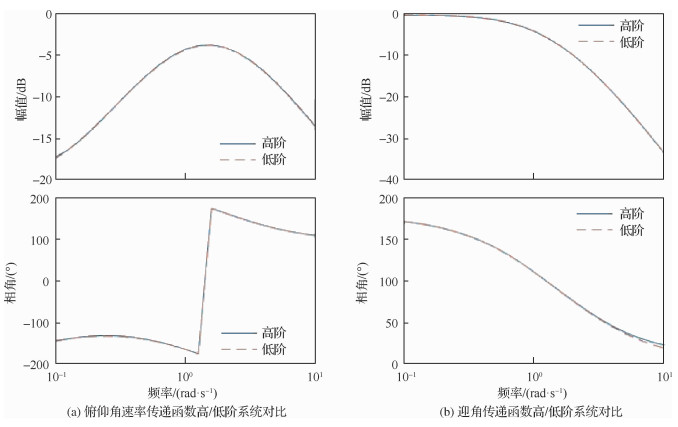图 3 高阶系统和低阶等效系统频率响应对比 Fig. 3 Comparison of frequency response between HOS and LOES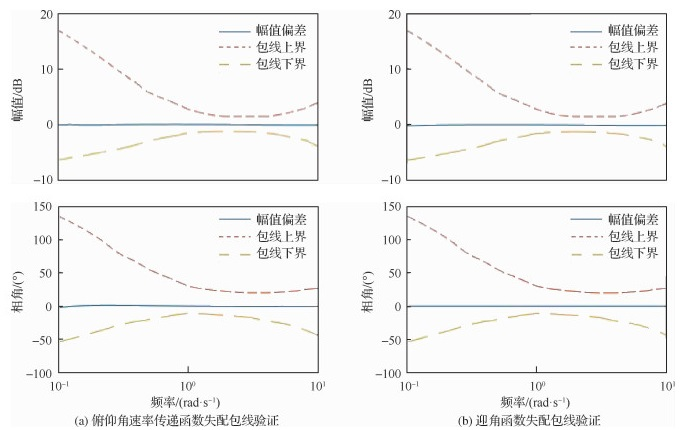图 4 频域等效拟配的失配包线验证 Fig. 4 Mismatched envelope verification in frequency domain equivalent matching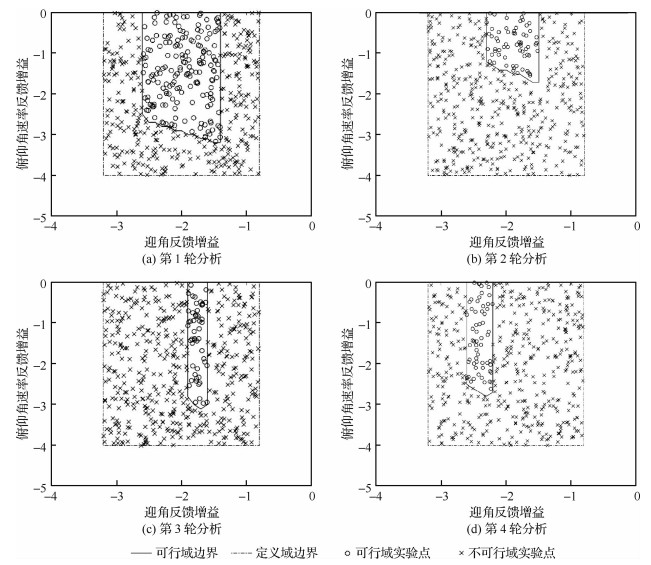图 5 控制律参数取值域内实验点分布 Fig. 5 Experimental points in control law parameter domain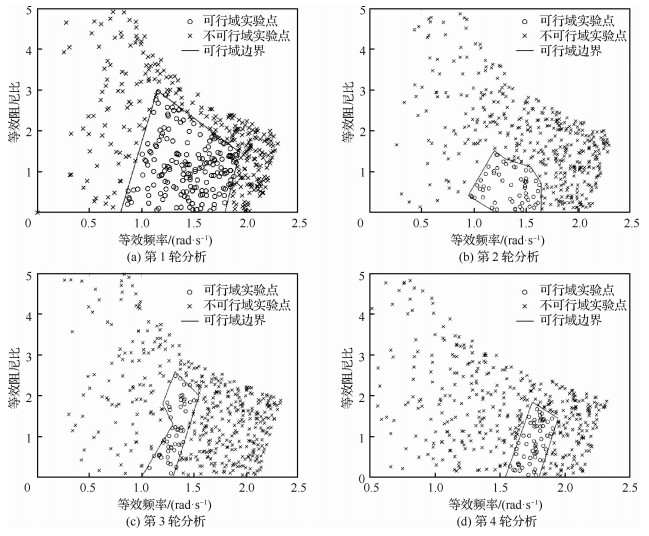图 6 实验点在底层指标取值域内的分布 Fig. 6 Experimental points in underlying indicator domain

 轮次 可行域内实验点数目 不可行域内实验点数目 重叠区域内实验点数目 错误率/% 1 177 323 10 2.00 2 55 445 5 1.00 3 51 449 4 0.80 4 59 441 4 0.80

4 结论

1) 关于采用阻尼频率特性作为指定飞行任务的底层评价指标时，本文的分析结果和军标MIL-F-8785C观点一致。

2) 本文提出的利用统计实验方法分析映射关系具有较好的通用性。

3) 本文提出的可行性分析流程具有良好的可实现性。

  冯振欣, 郭建国, 周军. 高超声速飞行器新型预设性能控制器设计[J]. 宇航学报, 2018, 39(6): 656-663. FENG Z X, GUO J G, ZHOU J. Novel prescribed performance controller design for a hypersonic vehicle[J]. Journal of Astronautics, 2018, 39(6): 656-663. (in Chinese) Cited By in Cnki (4) | Click to display the text  郁嘉, 杨鹏飞, 严德. 高超声速飞行器模型不确定性影响分析[J]. 航空学报, 2015, 36(1): 192-200. YU J, YANG P F, YAN D. Influence analysis of hypersonic flight vehicle model uncertainty[J]. Acta Aeronautica et Astronautica Sinica, 2015, 36(1): 192-200. (in Chinese) Cited By in Cnki (14) | Click to display the text  GREENE K M, KUNZ D L, COTTING M C. Toward a flying qualities standard for unmanned aircraft[C]//AIAA Atmospheric Flight Mechanics Conference. Reston, VA: AIAA, 2014.  GURUSWAMY G. Dynamic stability analysis of hypersonic transport during reentry[C]//Atmospheric Flight Mechanics Conference. Reston, VA: AIAA, 2016: 3374-3381.  HAYA-RAMOS R, PENIN L, PARIGINI C, et al. Flying qualities analysis for re-entry vehicles: Methodology and application[C]//AIAA Guidance, Navigation, and Control Conference. Reston, VA: AIAA, 2011.  陈柏屹, 刘燕斌, 雷灏, 等. 输入饱和及带宽限制下高超飞行器的闭环稳定边界研究[J]. 控制理论与应用, 2016, 33(11): 1508-1518. CHEN B Y, LIU Y B, LEI H, et al. Stability boundary analysis of hypersonic vehicle with control saturation and bandwidth limitation[J]. Control Theory & Applications, 2016, 33(11): 1508-1518. (in Chinese) Cited By in Cnki (5) | Click to display the text  MILLER R B, PACHTER M. Maneuvering flight control with actuator constraints[J]. Journal of Guidance Control & Dynamics, 2015, 20(4): 729-734. Click to display the text  LIU Y B, XIAO D B, LU Y P. Research on advanced flight control methods based on actuator constraints for elastic model of hypersonic vehicle[J]. Proceedings of the Institution of Mechanical Engineers, Part G:Journal of Aerospace Engineering, 2014, 228(9): 1627-1637. Click to display the text  陆宇平, 陈柏屹, 刘燕斌, 等. 吸气式高超声速飞行器考虑控制约束的设计优化[J]. 控制理论与应用, 2014, 31(12): 1695-1706. LU Y P, CHEN B Y, LIU Y B, et al. Control relevant design optimization for air-breathing hypersonic vehicle considering performance limitation[J]. Control Theory & Applications, 2014, 31(12): 1695-1706. (in Chinese) Cited By in Cnki (5) | Click to display the text  马卫华. 高超声速飞行器制导与控制性能评估方法[J]. 航天控制, 2012, 30(4): 7-12. MA W H. Research on evaluation method of guidance and control performance for hypersonic vehicle[J]. Aerospace Control, 2012, 30(4): 7-12. (in Chinese) Cited By in Cnki (13) | Click to display the text  杨琪琛, 宗群, 董琦. 高超声速飞行器再入控制与性能评估方法[J]. 信息与控制, 2017, 46(1): 33-40. YANG Q S, ZONG Q, DONG Q. Reentry control and performance evaluation method for hypersonic vehicle[J]. Information and Control, 2017, 46(1): 33-40. (in Chinese) Cited By in Cnki (13) | Click to display the text  顾杰, 张曙光, 杨帆, 等. 再入飞行器沉浮特性近似解析及应用[J]. 航空学报, 2017, 38(10): 38-49. GU J, ZHANG S G, YANG F, et al. Approximate analytical analysis for phugoid characteristic of reentry vehicles and its applications[J]. Acta Aeronautica et Astronautica Sinica, 2017, 38(10): 38-49. (in Chinese) Cited By in Cnki (1) | Click to display the text  CHEN X Q, HOU Z X, LIU J X, et al. Phugoid dynamic characteristic of hypersonic gliding vehicles[J]. Science China (Information Sciences), 2011, 54(3): 542-550. Click to display the text  SMARSLOK B P, CULLER A J, MAHADEVAN S. Error quantification and confidence assessment of aerothermal model predictions for hypersonic aircraft[C]//53rd AIAA/ASME/ASCE/AHS/ASC Structures, Structural Dynamics and Materials Conference. Reston, VA: AIAA, 2012.  HEBBAL A, BREVAULT L, BALESDENT M. Multi-objective optimization using deep gaussian processes: Application to aerospace vehicle Design[C]//AIAA SciTech Forum. Reston, VA: AIAA, 2019.  Flying Qualities of Piloted Airplanes: MIL-F-8785C[S]. Washington, D.C.: US: Department of Defense, 1980.  VIAVATTENE G, MOOJI E. Flying qualities and controllability of hypersonic spaceplanes[C]//AIAA Guidance, Navigation, and Control Conference. Reston, VA: AIAA, 2019.  周志华. 机器学习[M]. 2016. ZHOU Z H. Machine learning[M]. 1st ed. Beijing: Tsinghua University Press, 2016: 29-30. (in Chinese)  ZINNECKER A, SERRANI A, BOLENDER M A, et al. Combined reference governor and anti-windup design for constrained hypersonic vehicles models[C]//AIAA Guidance, Navigation, and Control Conference. Reston, VA: AIAA, 2009.  肖地波, 陆宇平, 刘燕斌, 等. 应用保护映射理论的高超声速飞行器自适应控制律设计[J]. 航空学报, 2015, 36(10): 3327-3337. XIAO D B, LU Y P, LIU Y B, et al. Adaptive control law design using guardian maps theory for hypersonic vehicles[J]. Acta Aeronautica et Astronautica Sinica, 2015, 36(10): 3327-3337. (in Chinese) Cited By in Cnki (9) | Click to display the text  王永革, 滕岩梅, 贾超华, 等. 应用泛函分析[M]. 第1版.北京: 北京航空航天大学出版社, 2012: 45-59. WANG Y G, TENG Y M, JIA C H, et al. Practical functional analysis[M]. 1st ed. Beijing: Beihang University Press, 2012: 45-59. (in Chinese)  ZONG Q, JI Y H, ZENG F L, et al. Output feedback back-stepping control for a generic hypersonic vehicle via small-gain theorem[J]. Aerospace Science and Technology, 2012, 23: 409-417. Click to display the text  BU X W, WU X Y, ZHANG R, et al. Tracking differentiator design for the robust backstepping control of a flexible air-breathing hypersonic vehicle[J]. Journal of the Franklin Institute, 2015, 352(4): 1739-1765. Click to display the text  戴华. 矩阵论[M]. 第1版.北京: 科学出版社, 2001: 189-193. DAI H. Matrix analysis[M]. 1st ed. Beijing: Science Press, 2001: 189-193. (in Chinese)  Flying Qualities of Piloted Vehicles: MIL-STD-1797[S]. Washington, D.C.: USAF, 1987-03.  FIELD E J, ROSSITTO K F, HODGKINSON J. Flying qualities applications of frequency responses identified from flight data[J]. Journal of Aircraft, 2004, 41(4): 711-720. Click to display the text  张勇, 陆宇平, 刘燕斌, 等. 高超声速飞行器控制一体化设计[J]. 航空动力学报, 2012, 27(12): 2724-2732. ZHANG Y, LU Y P, LIU Y B, et al. Control integrated design for hypersonic vehicle[J]. Journal of Aerospace Power, 2012, 27(12): 2724-2732. (in Chinese) Cited By in Cnki | Click to display the text  GROVES K P, SIGTHORSSON D O, SERRANI A. Reference command tracking for a linearized model of an air-breathing hypersonic vehicle[C]//AIAA Guidance, Navigation, and Control Conference and Exhibit. Reston, VA: AIAA, 2005.  BOLENDER M A, DOMAN D B. Nonlinear longitudinal dynamical model of an air-breathing hypersonic vehicle[J]. Journal of Spacecraft and Rockets, 2007, 44(2): 374-387. Click to display the text  张登辉, 马萍, 晁涛, 等. 高超声速飞行器制导控制系统性能评估[J]. 系统工程与电子技术, 2018, 40(8): 1811-1816. ZHANG D H, MA P, CHAO T, et al. Performance evaluation of guidance and control system for hypersonic vehicle[J]. Systems Engineering and Electronic, 2018, 40(8): 1811-1816. (in Chinese) Cited By in Cnki | Click to display the text
http://dx.doi.org/10.7527/S1000-6893.2019.23259

0

#### 文章信息

ZHU Hongxu, LIU Yanbin, CAO Rui, LU Yuping, TANG Jiajun, YI Chunlun

Feasibility analysis for underlying indictors in control performance evaluation of hypersonic vehicles

Acta Aeronautica et Astronautica Sinica, 2020, 41(3): 323259.
http://dx.doi.org/10.7527/S1000-6893.2019.23259